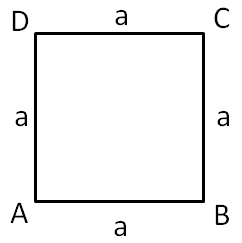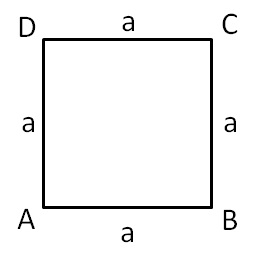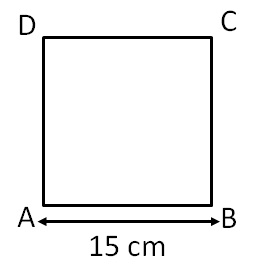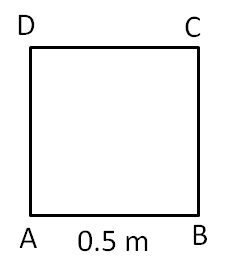Perimeter of Square

Chapter 10 Class 6 Mensuration
Concept wise

Let’s take a squareWe know that

In a square, all sides are equal

AB = BC = CD = AD

Let AB = a

So,

AB = BC = CD = AD = aNow,

Perimeter of square = Sum of all sides

= AB + BC + CD + AD

= a + a + a + a

= 4a

= 4 × Side of square

So,

Perimeter of square = 4a

= 4 × Side of square

Let’s solve some examples

#### Find perimeter of a square whose side is 15 cmGiven,

Side of square = a = 15 cm

So,

Perimeter of square = 4 × side of square

= 4a

= 4 × 15

= 60 cm

Thus, Perimeter of square is 60 cm

#### Find perimeter of a square whose side is 0.5 mGiven,

Side of square = a = 0.5 m

= 0.5 × 100 cm  (1m = 100 cm)

= 50 cm

So, Perimeter of square = 4 × Side of square

= 4a

= 4 × 50

= 200 cm

= 200/100 m         (1 cm =  1/100 m )

= 2 m

Learn in your speed, with individual attention - Teachoo Maths 1-on-1 Class